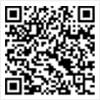# MBA联考《数学》模拟试题

MBA报名、考试、查分时间 免费短信提醒看不清楚，换张图片

【摘要】根据学员对MBA考研报考问题的反馈，同时为进一步加深大家对MBA考研相关信息的了解，环球网校老师为大家整理了“MBA联考《数学》模拟试题”，希望对大家有所帮助，环球网校将会及时为大家提供更多MBA考试信息，敬请关注。

1、 某中学从高中7个班中选出12名学生组成校代表队，参加市中学数学应用题竞赛活动，使代表中每班至少有1人参加的选法共有多少种?(462)

【思路1】剩下的5个分配到5个班级.c(5,7)

【思路2】C(6,11)=462

2、 在10个信箱中已有5个有信，甲、乙、丙三人各拿一封信，依次随便投入一信箱。求：

(1)甲、乙两人都投入空信箱的概率。

(2)丙投入空信箱的概率。

【思路】(1)A=甲投入空信箱，B=乙投入空信箱，P(AB)=C(1,5)*C(1,4)/(10*10)=1/5

(2)C=丙投入空信箱，

P(C)=P(C*AB) P(C* B) P(C*A ) P(C* )=(5*4*3 5*5*4 5*6*4 5*5*5)/1000=0.385

3、 设A是3阶矩阵，b1=(1,2,2)的转置阵，b2=(2,-2,1)的转置阵,b3=(-2,-1,2)的转置阵,满足Ab1=b1,Ab2=2b2,Ab3=3b3,求A.

【思路】可化简为A(b1,b2,b3)= (b1,b2,b3)

4、 已知P(A)=X,P(B)=2X,P(C)=3X且P(AB)=P(BC),求X的最大值.

【思路】P(BC)=P(AB)=P(A)=X

P(BC)=P(AB)小于等于P(A)=X

P(B C)=P(B) P(C)-P(BC)大于等于4X

4X小于等于1 ，X小于等于1/4

5、 在1至2000中随机取一个整数，求

(1)取到的整数不能被6和8整除的概率

(2)取到的整数不能被6或8整除的概率

【思路】设A=被6整除，B=被8整除;

P(B)=[2000/8]/2000=1/8=0.125;

P(A)=[2000/6]/2000=333/2000=0.1665;[2000/x]代表2000/x的整数部分;

(1)求1-P(AB);AB为A 、B的最小公倍数;

P(AB)=[2000/24]/2000=83/2000=0.0415;答案为1-0.0415=0.9585

(2)求1-P(A B);P(A B)=P(A) P(B)-P(AB)=0.25;答案为1-0.25=0.75.

6、已知f(xy)=f(x) f(y)且f’(1)=a,x≠0，求f’(x)=? (答案为a/x)

【思路1】原方程两边对Y进行求偏导

xf’(xy)=f’(y) 其中f’(xy)与f’(y)都是对y偏导数

xf’(x*1)=f’(1)=a 得 f’(x)=a/x

【思路2】当⊿x→0时，令x ⊿x=xz则z=(1 ⊿x/x)

={f[x(1 ⊿x/x)]-f(x)}/⊿x

=[f(x) f(1 ⊿x/x)-f(x)]/⊿x

=f(1 ⊿x/x)/⊿x =f’(1)/x=a/x

2020MBA考研预报名预计将于9月进行，初试预计会在12月21日左右，如果怕自己错过报名时间和考试时间的话，可以 免费预约短信提醒

|

## MBA最新文章推荐MBA各地入口iPhone版